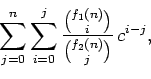# Some Double Sums Involving Ratios ofBinomial Coefficients ArisingFrom Urn Models

A1 Originalartikel i en vetenskaplig tidskrift (referentgranskad)

## Interna författare/redaktörer

 Stenlund, David
Publikationens författare: David Stenlund, James Gu Feng Wan
Förläggare: University of Waterloo
Publiceringsår: 2019
Tidskrift: Journal of Integer Sequences
Volym: 22

## Abstrakt

In this paper we discuss a class of double sums involving ratios of binomial coefficients. The sums are of the formwhere f1, f2 are functions of n. Such sums appear in the analyses of the Mabinogion urn and the Ehrenfest urn in probability. Using hypergeometric functions, we are able to simplify these sums, and in some cases express them in terms of the harmonic numbers.

## Nyckelord

Binomial identities, Hypergeometric functions, Urn models